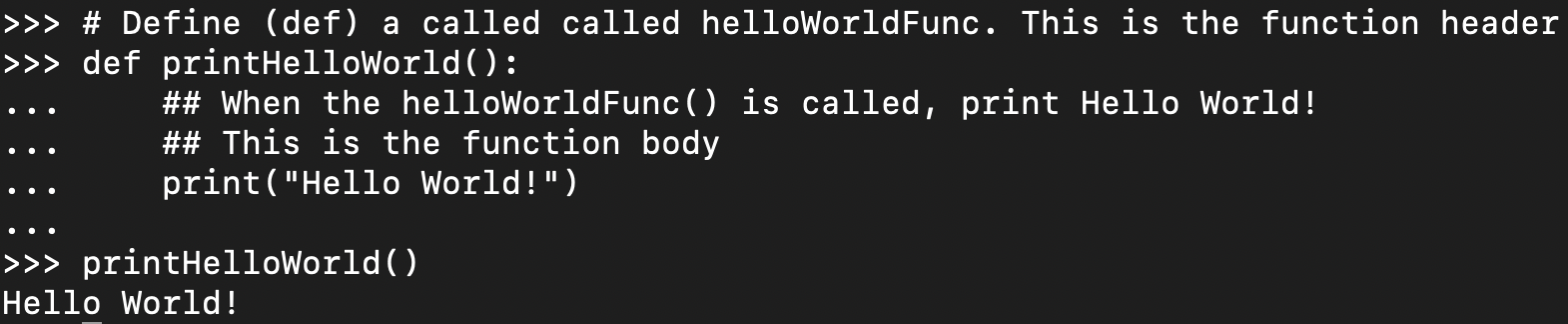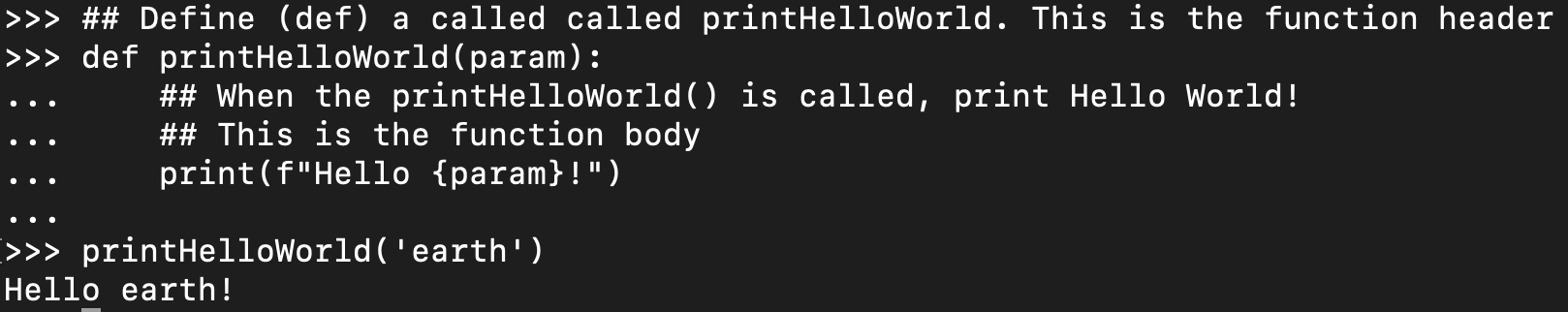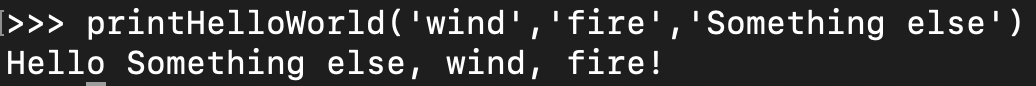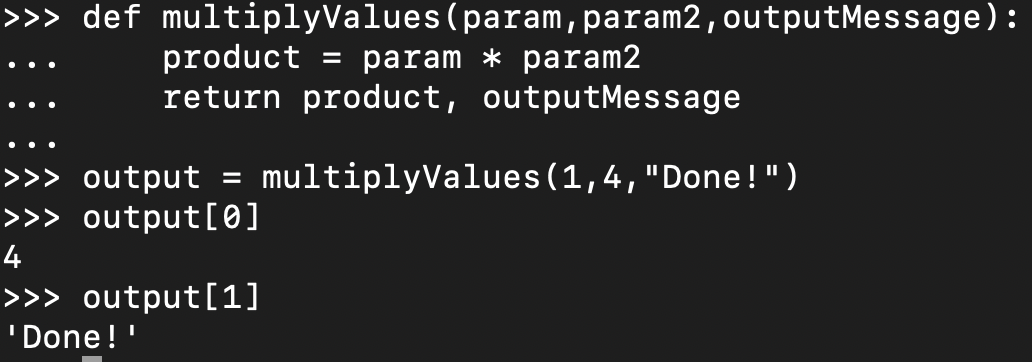## Getting Started: Python Functions for Newbies

Python is one of the most popular programming languages in the world. Talented developers can build just about anything in Python. To build great Python code, you must first understand Python functions. Functions start you down the path of creating modular code and reducing code duplication.

In this tutorial, you will learn how to get started with Python functions. You’ll learn what a Python function is, how to build function parameters, how to call them, function scope, and a whole lot more!

Let’s begin!

Table of Contents

## Prerequisites

This tutorial is hands-on and will include many different demos. If you intend to follow along, be sure you have the following:

• Python v3+ on any operating system. This tutorial will use Python v3.8.4.

## What is a Python Function?

When you begin writing code in just about any programming language, you’ll typically write it in one file as a single unit. You then execute the code in one large chunk. This approach may work at first, but ultimately, it will become unmanageable.

At some point, you realize you’re duplicating code when you really just need a way to call snippet of code when needed. At this point, you realize you need to learn functions and Python is no different.

In its simplest form, a Python function, like other language functions, is a block of code that Python only executes when called upon. It’s a reusable portion of code you can call upon in other parts of your Python script when needed.

Python functions also have optional inputs and outputs and allow you to modify the behavior of a function.

## Creating and Calling Your First Python Function

Let’s now get your hands dirty and start building your first Python function! To get started, open your favorite code editor and create (and save) a Python script called python_function_demo.py.

1. In the Python script, copy and paste the following code and save the script.

Python functions are declared or defined with the `def` keyword followed by the function name and a pair of parentheses with optional parameters, which you’ll learn later. This first line is called the function header.

Be sure to name functions by exactly what their purpose is. For example, in the code snippet below, a function name of `someFunction()` would work, but it doesn’t accurately describe what the function is actually doing.

Once you’ve defined the function header, you must then add the code the function should execute known as the function body.

Be sure to include four spaces of a tab for all code in the function body for Python to recognize that code is part of the function body.

``````## Define (def) a called called printHelloWorld. This is the function header
def printHelloWorld():
## When the printHelloWorld() is called, print Hello World!
## This is the function body
print("Hello World!")``````

2. Now, execute the script. Notice that Python essentially did nothing. It didn’t print `Hello world!` like you might have expected. In fact, it didn’t seem to do anything! Why? Because a function does not execute the code in the function body if you don’t call it first.

3. Next, add the `printHelloWorld()` line to make you script look like below and execute it again.

``````## Define (def) a called called printHelloWorld. This is the function header
def printHelloWorld():
## When the printHelloWorld() is called, print Hello World!
## This is the function body
print("Hello World!")
printHelloWorld()``````

You’ll see below that Python now returns the expected `Hello World!` text. It does this because you’ve now called or executed the `printHelloWorld()` function in the same script.Copy/pasting a function declaration and function call in a Python terminal and executing it

## Adding Python Function Parameters and Arguments

In the `printHelloWorld()` function example, you’re working with, this function has no inputs. You call it by its name, and Python executes it. But what if you need to change the behavior of the code inside the function or return a different output. You’ll do this by using a parameter.

You’ll commonly see the term ‘parameter’ and ‘argument’ used interchangeably. Technically, the parameter is the name (`param`), and the argument is the parameter’s value that’s passed to the function when called.

A parameter is a way to provide input to a function which the function can use when you call it. Using the tutorial example, add a parameter to the function called `param` by inserting it between the parentheses in the function header.

Then, replace `World` in the `print()` line with a placeholder for the `param` parameter, which will be the value of `param` when you call the function.

When you’re done, execute the script below.

``````## Define (def) a called called printHelloWorld. This is the function header
## This function has a single parameter
def printHelloWorld(param):
## When the printHelloWorld() is called, print Hello <whatever the parameter
## argument is>!
print(f"Hello {param}!")

printHelloWorld('earth')``````

The example function’s `print()` statement now uses Python string interpolation to specify a variable inside of a string.Demonstrating Python function parameters

### Adding Multiple Parameters to a Function

Using the same technique when defining a single function parameter, you can add as many as you’d like. Replace the example script with the following. Add as many comma-delimited parameters as you’d like. Once defined, you can then reference the parameter values inside of the function body.

Execute the example script below again, and you’ll notice that Python prints the values of each parameter you pass to the function.

``````## Define (def) a called called printHelloWorld. This is the function header
## This function has three parameters
def printHelloWorld(param,param2,param3):
## When the printHelloWorld() is called, print Hello... followed by the values
## of the parameters
print(f"Hello {param}, {param2}, {param3}!")

printHelloWorld('earth','wind','fire')``````

Parameter order is important in Python functions. When you pass arguments to a function parameter, Python will match each argument to a parameter in the same order as you define the parameters in the function header.

### Default Function Arguments

If you find yourself passing the same argument to a function parameter repeatedly, defining a default argument for that parameter may be wise. If, for example, you’d like to ensure the `param3` parameter in the example function will have the value `Earth` by default, you’d define the value like below.

``````## This function has three parameters with on default argument
def printHelloWorld(param,param2,param3='Earth'):
print(f"Hello {param}, {param2}, {param3}!")

printHelloWorld('wind','fire')``````

If at any point, you’d like to override that default argument value, you’d then simply specify `param3`‘s argument while calling the function like below.

``printHelloWorld('wind','fire','Something else')``Overriding the function default argument value

## Function Scope

Like many other programming languages, Python has a concept called scope. Scope refers to where variables and other information are stored. For example, when you define a Python variable in a script and call it in the same script (not in a function), Python can find that variable since it’s “local” to where you defined it.

``````variable = 'foo'

## This works because variable was defined and referenced in the same scope
print(variable)``````

This tutorial will not cover scope in-depth but it’s important to understand when building functions.

Inside of the tutorial example function, you have three variables representing the argument value of three parameters; `param`, `param2`, and `param3`. These three variables inside of the function are called local variables. They are called ‘local’ variables because they are local to the function. Thus, they are scoped to the function.

To demonstrate this concept, add the same `print()` statement right below the line in the example script that’s in the function body and execute the script.

``````def printHelloWorld(param,param2,param3='Earth'):
print(f"Hello {param}, {param2}, {param3}!")

printHelloWorld('wind','fire')

print(f"Hello {param}, {param2}, {param3}!")``````

Notice below that, when referenced inside the function, the function prints the arguments as expected, but when you try to reference those same arguments outside of the function, it fails.

Python cannot see the `param`, `param2` and `param3` variables outside of the local scope of the function. These variables simply don’t exist in the script’s scope.Function prints the argument

## Returning Output with the Python Function Return Statement

So far in this tutorial, the function you’ve been working with has technically been returning something. You’ve been using the `print()` statement to return some text to the terminal. Using the `print()` statement works great if you need to inspect the output.

But when you need your function to return other types of Python objects, it’s time to use the `return` statement. If you need to assign the return value of a function to a variable and use it somewhere else, you must use the `return` statement.

To demonstrate the Python function return statement, create another function called `multipleValues()` and call it like below and run it. This function, given two values as parameters, multiplies those arguments together.

``````def multiplyValues(param,param2):
param * param2

multiplyValues(1,4)``````

When you run this script, you’ll notice no output is returned, which may lead you to believe Python didn’t execute the function, but it did. The function just didn’t return anything because you didn’t use the `return` statement.

When code like `1 * 4` returns a value outside of a function, but that same code inside a function seemingly doesn’t return anything without a `return` statement, that output is sometimes called implicit.

Now, include the Python function `return` statement as shown below, and you’ll see the function returns the product of `1` and `4`.

``````def multiplyValues(param,param2):
return param * param2

multiplyValues(1,4)``````

At this point, you can use the output of `multiplyValues()` to perform other mathematical tasks. This task wouldn’t be possible if you were using the `print()` statement.

``````product = multiplyValues(1,4)
product * 2``````Product Multiply Values

When you use the Python return statement to return output from a function, the value returned is sometimes called explicit output.

### Returning Multiple Values

Similarly, if you need to return multiples values from a Python function, you can do so by including one or more comma-delimited values after the Python function `return` statement.

For example, perhaps you’d like to create a function that multiplies two numbers and then returns a different message. To do so, you could assign the variable `product` to the product of the two values and then return the product and message as one like below.

``````def multiplyValues(param,param2,outputMessage):
product = param * param2
return product, outputMessage

multiplyValues(1,4,"Done!")``````Assign the Variable Product

When you return multiple values in a function, Python returns them as a Python tuple which you can then reference individual elements of like below.

``````def multiplyValues(param,param2,outputMessage):
product = param * param2
return product, outputMessage

output = multiplyValues(1,4,"Done!")
output
output``````return multiple values in a function

## Conclusion

You should now have a foundational understanding of Python functions and using the Python function return statement. But, this tutorial has just scratched the surface of what’s possible with Python functions.

How do you plan to use your newfound Python function knowledge?

## Subscribe to Stay in Touch

### Never miss out on your favorite ATA posts and our latest announcements!

Looks like you're offline!